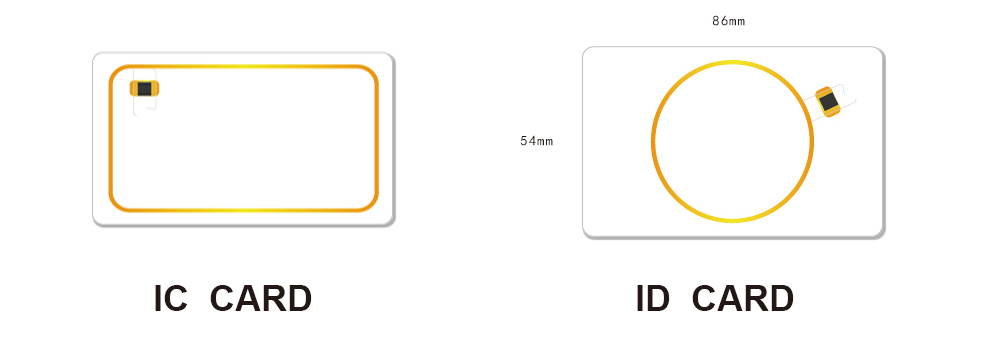Common formats of ID card and IC card internal code May 30, 2021

Abstract: In the production of smart IC cards, in order to facilitate the use and query of the system, most systems need to laser engrave the card's UID (commonly known as internal code) on the card surface. The following are some common internal code formats under analysis. Such as: Mifare® 1k S50 card, sector 0, block 0 data: 2A83155EE288040047C129D24D000607

Normally . we can see clear like below antenna , IC card mainly is 13.56Mhz contactless rfid card and rectangle shape antenna .  and ID card mainly is 125khz rfid chip card such as EM4200 cards ,T5577 cards ,etc .they will round shape antenna .Common format of IC card (13.56MHz HF Cards both reading and rewriting ) internal code:

In the production process of smart IC cards, in order to facilitate the use and query of the system, most systems need to laser engrave the card's UID (commonly known as internal code) on the card surface. The following are some common internal code formats under analysis.

For example: data of block 0 of sector 0 of Mifare 1k S50 card:

2A83155EE288040047C129D24D000607

1. The positive code is "2A83155E", which is the first 4 bytes of block 0 in sector 0,

A total of 8 numbers or letters.

2. The inverted code is "5E15832A", that is, the first 4 bytes of block 0 in sector 0 are read backwards.

A total of 8 numbers or letters.

ABA code (decimal):

1. Taking the positive code is the decimal number converted from the Manchester code. The calculation result is "0713233758" according to the example.

A total of 10 numbers.

2. The inverted code is the decimal number converted from the inverted code of Manchester code. According to the example, the calculation result is "1578468138".

A total of 10 numbers.

Common format of ID card internal code

Manchester code is hexadecimal, ABA code and Wiegand code are both decimal.

Manchester internal code of ID card = version code + customer code + ID code

Example: 125533FFFF, the version code is "1", the customer code is "2", and the ID code (8 numbers or letters) is "5533FFFF".

ABA (8H): ID code (8 numbers or letters, according to the example is "5533FFFF") converted to decimal,

According to the example, the calculation result is "1429471231", a total of 10 numbers.

ABA (6H): ID code (the last 6 digits or letters, according to the example is "33FFFF") converted to decimal,

According to the example, the calculation result is "03407871", a total of 8 numbers.

ABA (4H): ID code (the last 4 digits or letters, according to the example is "FFFF") converted to decimal,

According to the example, the calculation result is "65535", a total of 5 numbers.

WG26 (2H+4H): ID code (the 6th and 5th digits or letters from the bottom + the last 4 digits or letters, according to the example is "33+FFFF")

Converted to decimal system, the calculation result according to the example is "051,65535", a total of 8 digits.

WG34 (4H+4H): ID code (8th, 7th, 6th, 5th digits or letters from the bottom + last 4 digits or letters, according to the example is "5533+FFFF")

Converted to decimal system, the calculation result according to the example is "21811,65535", a total of 10 digits.

Statement : Pictures above only show the product ,the intellectual property rights of the logo owned by their trademark

Categories
New Blog
Tags